Since its release last week, I've been playing quite a bit of Fallout 4. There's an interesting mini-game (which was in previous iterations as well) for "hacking" computer terminals, where you must guess the passcode on a list of possibilities with a limited number of guesses. Each failed guess provides the number of correct letters (in both value and position) in that particular word, but not which letters were correct, allowing you to deduce the correct passcode similarly to the game "Mastermind." A natural question is, "what is the best strategy for identifying the correct passcode?" We'll ignore the possibility of dud removal and guess resets (which exist to simplify it a bit in game) for the analysis.

Reformulating this as a probability question offers a framework to design the best strategy. First, some definitions:denotes the number of words,denotes the correct word, and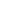denotes a word on the list (in some consistent order). A simple approach suggests that we want to use the maximum likelihood (ML) estimate ofto choose the next word based on all the words guessed so far and their results: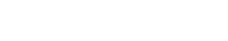However, for the first word, the probability prior is uniform--each word is equally likely. This might seem like the end of the line, so just pick the first word randomly (or always pick the first one on the list, for instance). However, future guesses depend on what this first guess tells us, so we'd be better off with an estimate which maximizes the mutual information between the guess and the unknown password. Using the concept of entropy (which I've discussed briefly before), we can formalize the notion of "mutual information" into a mathematical definition: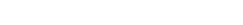. In this sense, "information" is what you gain by making an observation, and it is measured by how it affects the possible states for a latent variable to take. For more compact notation, let's define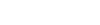as the "result" random variable for a particular word, telling us how many letters matched, taking values, where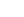is the length of words in the current puzzle. Then, we can change our selection criteria to pick the maximum mutual information: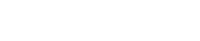But, we haven't talked about what "conditional entropy" might mean, so it's not yet clear how to calculate, apart from it being the entropy after observing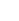's value. Conditional entropy is distinct from conditional probability in a subtle way: conditional probability is based on a specific observation, such as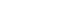, but conditional entropy is based on all possible observations and reflects how many possible system configurations there are after making an observation, regardless of what its value is. It's a sum of the resulting entropy after each possible observation, weighted by the probability of that observation happening: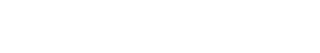As an example, let's consider a puzzle with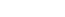and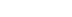. We know that. If we define the similarity functionto be the number of letters that match in place and value for two words, and we define the group of sets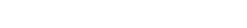as the candidate sets, then we can find the probability distribution forby counting,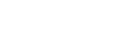As a sanity check, we know that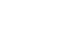because there are no duplicates, and therefore this equation matches our intuition for the probability of each word being an exact match. With the definition ofin hand, all that remains is finding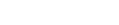, but luckily our definition for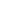has already solved this problem! If, then we know that the true solution is uniformly distributed in, so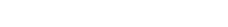Finding the best guess is as simple as enumeratingand then finding thewhich produces the minimum conditional entropy. For subsequent guesses, we simply augment the definition for the candidate sets by further stipulating that set members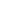must also be in the observed set for all previous iterations. This is equivalent to taking the set intersection, but the notation gets even messier than we have so far, so I won't list all the details here.

All that said, this is more of an interesting theoretical observation than a practical one. Counting all of the sets by hand generally takes longer than a simpler strategy, so it is not well suited for human use (I believe it isoperations for each guess), although a computer can do it effectively. Personally, I just go through and find all the emoticons to remove duds and then find a word that has one or two overlaps with others for my first guess, and the field narrows down very quickly.

Beyond its appearance in a Fallout 4 mini-game, the concept of "maximum mutual information" estimation has broad scientific applications. The most notable in my mind is in machine learning, where MMI is used for training classifiers, in particular, Hidden Markov Models (HMMs) such as those used in speech recognition. Given reasonable probability distributions, MMI estimates are able to handle situations where ML estimates appear ambiguous, and as such they are able to be used for "discriminative training." Typically, an HMM training algorithm would receive labeled examples of each case and learn their statistics only. However, a discriminative trainer can also consider the labeled examples of other cases in order to improve classification when categories are very similar but semantically distinct.